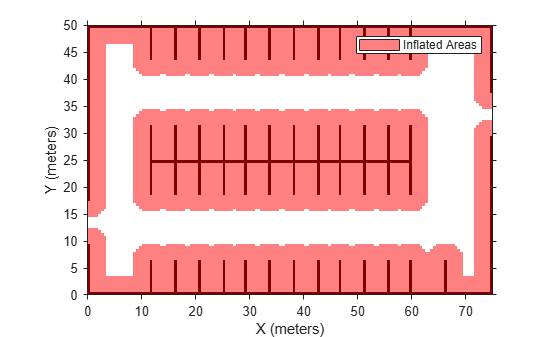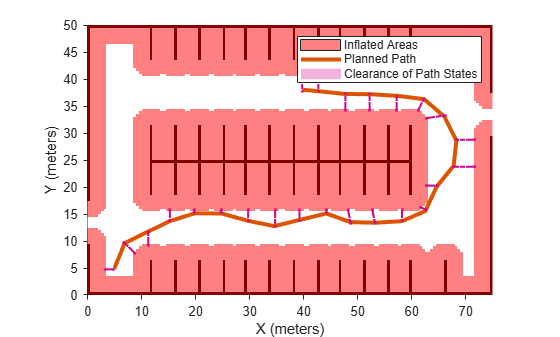# clearance

Minimum clearance of path

## Syntax

``clearance(pathMetricsObj)``
``clearance(pathMetricsObj,'Type','states')``

## Description

example

````clearance(pathMetricsObj)` returns the minimum clearance of the path. Clearance is measured as the minimum distance between grid cell centers of states on the path and obstacles in the specified map environment. NoteThe computed clearance is accurate up to `sqrt(2)` times grid map cell size. ```
````clearance(pathMetricsObj,'Type','states')` returns the set of minimum distances for each state of the path, in the form of an n-by-1 vector, where n is the number of states.```

## Examples

collapse all

Compute smoothness, clearance, and validity of a planned path based on a set of poses and the associated map environment.

Load and Assign Map to State Validator

Create an occupancy map from an example map and set the map resolution.

```load exampleMaps.mat; % simpleMap mapResolution = 1; % cells/meter map = occupancyMap(simpleMap,mapResolution);```

Create a Dubins state space.

`statespace = stateSpaceDubins;`

Create a state validator based on occupancy map to store the parameters and states in the Dubins state space.

`statevalidator = validatorOccupancyMap(statespace);`

Assign the map to the validator.

`statevalidator.Map = map;`

Set the validation distance for the validator.

`statevalidator.ValidationDistance = 0.01;`

Update the state space bounds to be the same as the map limits.

`statespace.StateBounds = [map.XWorldLimits;map.YWorldLimits;[-pi pi]];`

Plan Path

Create an RRT* path planner and allow further optimization.

```planner = plannerRRTStar(statespace,statevalidator); planner.ContinueAfterGoalReached = true;```

Reduce the maximum number of iterations and increase the maximum connection distance.

```planner.MaxIterations = 2500; planner.MaxConnectionDistance = 0.3;```

Define start and goal states for the path planner as `[`x, y, theta`]` vectors. x and y are the Cartesian coordinates, and theta is the orientation angle.

```start = [2.5, 2.5, 0]; % [meters, meters, radians] goal = [22.5, 8.75, 0];```

Plan a path from the start state to the goal state. The plan function returns a `navPath` object.

```rng(100,'twister') % repeatable result [path,solutionInfo] = plan(planner,start,goal);```

Compute and Visualize Path Metrics

Create a path metrics object.

`pathMetricsObj = pathmetrics(path,statevalidator);`

Check path validity. The result is `1 (true)` if the planned path is obstacle free. `0 (false)` indicates an invalid path.

`isPathValid(pathMetricsObj)`
```ans = logical 1 ```

Calculate the minimum clearance of the path.

`clearance(pathMetricsObj)`
```ans = 1 ```

Evaluate the smoothness of the path. Values close to `0` indicate a smoother path. Straight-line paths return a value of `0`.

`smoothness(pathMetricsObj)`
```ans = 1.7453 ```

Visualize the minimum clearance of the path.

```show(pathMetricsObj) legend('Planned Path','Minimum Clearance')```Plan a vehicle path through a parking lot using the RRT* algorithm. Compute and visualize the smoothness, clearance, and validity of the planned path.

Load and Assign Map to State Validator

Load a costmap of a parking lot. Plot the costmap to see the parking lot and the inflated areas that the vehicle should avoid.

```load parkingLotCostmap.mat; costmap = parkingLotCostmap; plot(costmap) xlabel('X (meters)') ylabel('Y (meters)')```Create a `stateSpaceDubins` object and increase the minimum turing radius to `4` meters.

```statespace = stateSpaceDubins; statespace.MinTurningRadius = 4; % meters```

Create a `validatorVehicleCostmap` object using the created state space.

`statevalidator = validatorVehicleCostmap(statespace);`

Assign the parking lot costmap to the state validator object.

`statevalidator.Map = costmap;`

Plan Path

Define start and goal poses for the vehicle as [`x`, `y`, `Θ`] vectors. World units for the (`x`,`y`) locations are in meters. World units for the orientation angles `Θ` are in degrees.

```startPose = [5, 5, 90]; % [meters, meters, degrees] goalPose = [40, 38, 180]; % [meters, meters, degrees]```

Use a `pathPlannerRRT` (Automated Driving Toolbox) object and the `plan` (Automated Driving Toolbox) function to plan the vehicle path from the start pose to the goal pose.

```planner = pathPlannerRRT(costmap); refPath = plan(planner,startPose,goalPose);```

Interpolate along the path at every one meter. Convert the orientation angles from degrees to radians.

```poses = zeros(size(refPath.PathSegments,2)+1,3); poses(1,:) = refPath.StartPose; for i = 1:size(refPath.PathSegments,2) poses(i+1,:) = refPath.PathSegments(i).GoalPose; end poses(:,3) = deg2rad(poses(:,3));```

Create a `navPath` object using the Dubins state space object and the states specified by `poses`.

`path = navPath(statespace,poses);`

Compute and Visualize Path Metrics

Create a `pathmetrics` object.

`pathMetricsObj = pathmetrics(path,statevalidator);`

Check path validity. The result is `1` (`true`) if the planned path is obstacle free. `0` (`false`) indicates an invalid path.

`isPathValid(pathMetricsObj)`
```ans = logical 1 ```

Compute and visualize the minimum `clearance` of the path.

`clearance(pathMetricsObj)`
```ans = 0.5000 ```
```show(pathMetricsObj) legend('Inflated Areas','Planned Path','Minimum Clearance') xlabel('X (meters)') ylabel('Y (meters)')```Compute and visualize the `smoothness` of the path. Values close to `0` indicate a smoother path. Straight-line paths return a value of `0`.

`smoothness(pathMetricsObj)`
```ans = 0.0842 ```
```show(pathMetricsObj,'Metrics',{'Smoothness'}) legend('Inflated Areas','Path Smoothness') xlabel('X (meters)') ylabel('Y (meters)')```Visualize the clearance for each state of the path.

```show(pathMetricsObj,'Metrics',{'StatesClearance'}) legend('Inflated Areas','Planned Path','Clearance of Path States') xlabel('X (meters)') ylabel('Y (meters)')```## Input Arguments

collapse all

Information for path metrics, specified as a `pathmetrics` object.